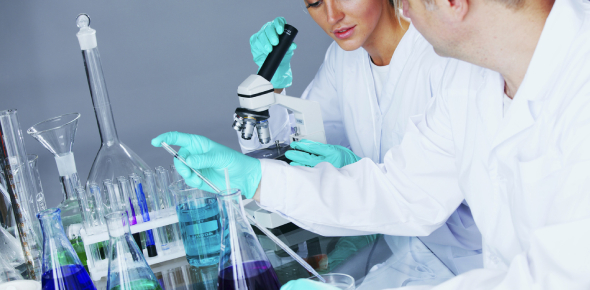# Year 11 Chemistry Quiz 1

9 Questions | Total Attempts: 7105SettingsIsotopes, Lewis diagrams, naming compounds, balancing equations

• 1.
How many neutrons are in potassium-40?
• A.

19

• B.

40

• C.

21

• D.

27

• 2.
What is the electron configuration for Sulphur?
• A.

(2, 8, 7)

• B.

(2, 6, 5)

• C.

(2, 8, 6)

• D.

(2, 6, 8)

• 3.
What are the coefficients for the following equation when it is balanced? ___SiO2 + ___HF  -->   ___SiF4  +  ___H2O
• A.

2, 3, 2, 2

• B.

1, 4, 1, 2

• C.

2, 4, 1, 3

• D.

1, 3, 2, 3

• 4.
How is a mixture different from a compound?
• A.

Particles of a mixture are combined chemically

• B.

Components of a mixture can be separated by physical means

• C.

Components of a mixture can only be seperated chemically

• D.

Composition of a mixture may be constant

• 5.
If a certain mixture is homogeneous, you would properly conclude that the physical properties and the composition are:
• A.

Vary smoothly from top to bottom of the sample

• B.

Are different from one part of the sample to another

• C.

Are the same in every small volume element of the sample

• D.

None of these

• 6.
What is the most correct Lewis Diagram for Helium?
• A.
• B.
• C.
• D.
• 7.
Name this compound: Hint: this is reaction between a non-metal and a non-metal
• A.

Nitrogen oxide

• B.

Dinitrogen tetroxide

• C.

Tetraoxygen dinitride

• D.

Oxygen nitride

• 8.
What is the name for the compound CuOH?
• A.

Copper hydroxide

• B.

Copper(I) hydrogen oxide

• C.

Copper(I) hydroxide

• D.

Copper hydrogen oxide

• 9.
Balance the following: ___BF3 +  ___Li2SO3  +  ___B2(SO3)3 + ___LiF What will the coefficients be for the balanced reaction?
• A.

2, 4, 1, 5

• B.

1, 2, 1, 3

• C.

2, 3, 1, 6

• D.

1, 3, 2, 6

Related TopicsBack to top